# 7.4 The other trigonometric functions  (Page 8/14)

 Page 8 / 14

Find the area of the sector of a circle with diameter 32 feet and an angle of $\text{\hspace{0.17em}}\frac{3\pi }{5}\text{\hspace{0.17em}}$ radians.

For the following exercises, find the angle between $\text{\hspace{0.17em}}0°\text{\hspace{0.17em}}$ and $\text{\hspace{0.17em}}\text{360°}\text{\hspace{0.17em}}$ that is coterminal with the given angle.

$420°$

$60°$

$-80°$

For the following exercises, find the angle between 0 and $\text{\hspace{0.17em}}2\pi \text{\hspace{0.17em}}$ in radians that is coterminal with the given angle.

$-\text{\hspace{0.17em}}\frac{20\pi }{11}$

$\frac{2\pi }{11}$

$\frac{14\pi }{5}$

For the following exercises, draw the angle provided in standard position on the Cartesian plane.

$-210°$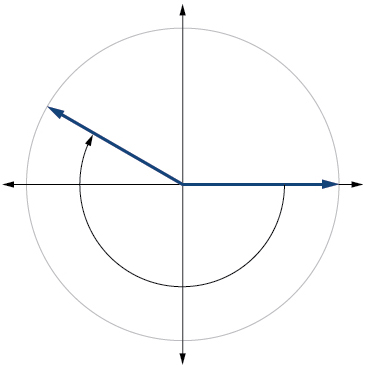$75°$

$\frac{5\pi }{4}$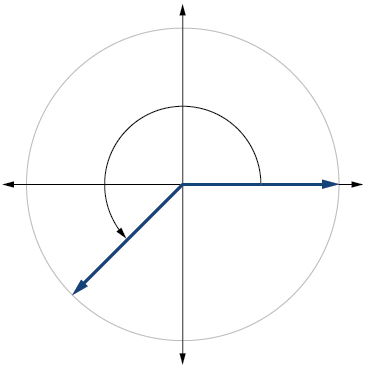$-\frac{\pi }{3}$

Find the linear speed of a point on the equator of the earth if the earth has a radius of 3,960 miles and the earth rotates on its axis every 24 hours. Express answer in miles per hour. Round to the nearest hundredth.

1036.73 miles per hour

A car wheel with a diameter of 18 inches spins at the rate of 10 revolutions per second. What is the car's speed in miles per hour? Round to the nearest hundredth.

## Right Triangle Trigonometry

For the following exercises, use side lengths to evaluate.

$\mathrm{cos}\text{\hspace{0.17em}}\frac{\pi }{4}$

$\frac{\sqrt{2}}{2}$

$\mathrm{cot}\text{\hspace{0.17em}}\frac{\pi }{3}$

$\mathrm{tan}\text{\hspace{0.17em}}\frac{\pi }{6}$

$\frac{\sqrt{3}}{3}$

$\mathrm{cos}\left(\frac{\pi }{2}\right)=\mathrm{sin}\left(___°\right)$

$\mathrm{csc}\left(18°\right)=\mathrm{sec}\left(___°\right)$

$72°$

For the following exercises, use the given information to find the lengths of the other two sides of the right triangle.

$\mathrm{cos}\text{\hspace{0.17em}}B=\frac{3}{5},a=6$

$\mathrm{tan}\text{\hspace{0.17em}}A=\frac{5}{9},b=6$

$a=\frac{10}{3},c=\frac{2\sqrt{106}}{3}$

For the following exercises, use [link] to evaluate each trigonometric function.

$\mathrm{tan}\text{\hspace{0.17em}}B$

$\frac{6}{11}$

For the following exercises, solve for the unknown sides of the given triangle.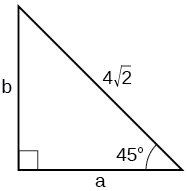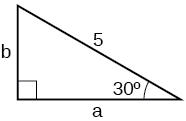$a=\frac{5\sqrt{3}}{2},b=\frac{5}{2}$

A 15-ft ladder leans against a building so that the angle between the ground and the ladder is $\text{\hspace{0.17em}}70°.\text{\hspace{0.17em}}$ How high does the ladder reach up the side of the building? Find the answer to four decimal places.

The angle of elevation to the top of a building in Baltimore is found to be 4 degrees from the ground at a distance of 1 mile from the base of the building. Using this information, find the height of the building. Find the answer to four decimal places.

369.2136 ft

## Unit Circle

Find the exact value of $\text{\hspace{0.17em}}\mathrm{sin}\text{\hspace{0.17em}}\frac{\pi }{3}.$

Find the exact value of $\text{\hspace{0.17em}}\mathrm{cos}\text{\hspace{0.17em}}\frac{\pi }{4}.$

$\frac{\sqrt{2}}{2}$

Find the exact value of $\text{\hspace{0.17em}}\mathrm{cos}\text{\hspace{0.17em}}\pi .$

State the reference angle for $\text{\hspace{0.17em}}300°.\text{\hspace{0.17em}}$

$60°$

State the reference angle for $\text{\hspace{0.17em}}\frac{3\pi }{4}.$

Compute cosine of $\text{\hspace{0.17em}}330°.$

$\frac{\sqrt{3}}{2}$

Compute sine of $\text{\hspace{0.17em}}\frac{5\pi }{4}.$

State the domain of the sine and cosine functions.

all real numbers

State the range of the sine and cosine functions.

## The Other Trigonometric Functions

For the following exercises, find the exact value of the given expression.

$\mathrm{cos}\text{\hspace{0.17em}}\frac{\pi }{6}$

$\frac{\sqrt{3}}{2}$

$\mathrm{tan}\text{\hspace{0.17em}}\frac{\pi }{4}$

$\mathrm{csc}\text{\hspace{0.17em}}\frac{\pi }{3}$

$\frac{2\sqrt{3}}{3}$

$\mathrm{sec}\text{\hspace{0.17em}}\frac{\pi }{4}$

For the following exercises, use reference angles to evaluate the given expression.

$\mathrm{sec}\text{\hspace{0.17em}}\frac{11\pi }{3}$

2

$\mathrm{sec}\text{\hspace{0.17em}}315°$

If $\text{\hspace{0.17em}}\mathrm{sec}\left(t\right)=-2.5,$ what is the $\text{\hspace{0.17em}}\text{sec}\left(-t\right)?$

–2.5

If $\text{\hspace{0.17em}}\text{tan}\left(t\right)=-0.6,$ what is the $\text{\hspace{0.17em}}\text{tan}\left(-t\right)?$

If $\text{\hspace{0.17em}}\text{tan}\left(t\right)=\frac{1}{3},$ find $\text{\hspace{0.17em}}\text{tan}\left(t-\pi \right).$

$\frac{1}{3}$

If $\text{\hspace{0.17em}}\text{cos}\left(t\right)=\frac{\sqrt{2}}{2},$ find $\text{\hspace{0.17em}}\text{sin}\left(t+2\pi \right).$

Which trigonometric functions are even?

cosine, secant

Which trigonometric functions are odd?

## Chapter practice test

Convert $\text{\hspace{0.17em}}\frac{5\pi }{6}\text{\hspace{0.17em}}$ radians to degrees.

$150°$

Convert $\text{\hspace{0.17em}}-620°\text{\hspace{0.17em}}$ to radians.

Find the length of a circular arc with a radius 12 centimeters subtended by the central angle of $\text{\hspace{0.17em}}30°.$

6.283 centimeters

Find the area of the sector with radius of 8 feet and an angle of $\text{\hspace{0.17em}}\frac{5\pi }{4}\text{\hspace{0.17em}}$ radians.

Find the angle between $\text{\hspace{0.17em}}0°\text{\hspace{0.17em}}$ and $\text{\hspace{0.17em}}\text{360°}\text{\hspace{0.17em}}$ that is coterminal with $\text{\hspace{0.17em}}375°.$

$15°$

Find the angle between 0 and $\text{\hspace{0.17em}}2\pi \text{\hspace{0.17em}}$ in radians that is coterminal with $\text{\hspace{0.17em}}-\frac{4\pi }{7}.$

Draw the angle $\text{\hspace{0.17em}}315°\text{\hspace{0.17em}}$ in standard position on the Cartesian plane.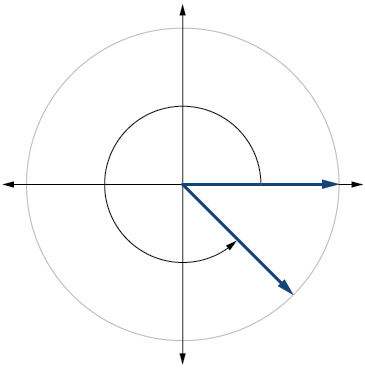Draw the angle $\text{\hspace{0.17em}}-\frac{\pi }{6}\text{\hspace{0.17em}}$ in standard position on the Cartesian plane.

A carnival has a Ferris wheel with a diameter of 80 feet. The time for the Ferris wheel to make one revolution is 75 seconds. What is the linear speed in feet per second of a point on the Ferris wheel? What is the angular speed in radians per second?

3.351 feet per second, $\text{\hspace{0.17em}}\frac{2\pi }{75}\text{\hspace{0.17em}}$ radians per second

Find the missing sides of the triangle $\text{\hspace{0.17em}}ABC:\mathrm{sin}\text{\hspace{0.17em}}B=\frac{3}{4},c=12.$

Find the missing sides of the triangle.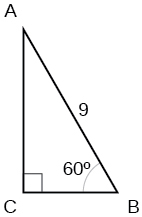$a=\frac{9}{2},b=\frac{9\sqrt{3}}{2}$

The angle of elevation to the top of a building in Chicago is found to be 9 degrees from the ground at a distance of 2000 feet from the base of the building. Using this information, find the height of the building.

Find the exact value of $\text{\hspace{0.17em}}\mathrm{sin}\text{\hspace{0.17em}}\frac{\pi }{6}.$

$\frac{1}{2}$

Compute sine of $\text{\hspace{0.17em}}240°.$

State the domain of the sine and cosine functions.

real numbers

State the range of the sine and cosine functions.

Find the exact value of $\text{\hspace{0.17em}}\mathrm{cot}\text{\hspace{0.17em}}\frac{\pi }{4}.$

1

Find the exact value of $\text{\hspace{0.17em}}\mathrm{tan}\text{\hspace{0.17em}}\frac{\pi }{3}.$

Use reference angles to evaluate $\text{\hspace{0.17em}}\mathrm{csc}\text{\hspace{0.17em}}\frac{7\pi }{4}.$

$\text{\hspace{0.17em}}-\sqrt{2}$

Use reference angles to evaluate $\text{\hspace{0.17em}}\mathrm{tan}\text{\hspace{0.17em}}210°.$

If $\text{\hspace{0.17em}}\text{csc}\text{\hspace{0.17em}}t=0.68,$ what is the $\text{\hspace{0.17em}}\text{csc}\left(-t\right)?$

–0.68

If $\text{\hspace{0.17em}}\text{cos}\text{\hspace{0.17em}}t=\frac{\sqrt{3}}{2},$ find $\text{\hspace{0.17em}}\text{cos}\left(t-2\pi \right).$

Find the missing angle: $\text{\hspace{0.17em}}\mathrm{cos}\left(\frac{\pi }{6}\right)=\mathrm{sin}\left(___\right)$

$\frac{\pi }{3}$

the third and the seventh terms of a G.P are 81 and 16, find the first and fifth terms.
if a=3, b =4 and c=5 find the six trigonometric value sin
pls how do I factorize x⁴+x³-7x²-x+6=0
in a function the input value is called
how do I test for values on the number line
if a=4 b=4 then a+b=
a+b+2ab
Kin
commulative principle
a+b= 4+4=8
Mimi
If a=4 and b=4 then we add the value of a and b i.e a+b=4+4=8.
Tariq
what are examples of natural number
an equation for the line that goes through the point (-1,12) and has a slope of 2,3
3y=-9x+25
Ishaq
show that the set of natural numberdoes not from agroup with addition or multiplication butit forms aseni group with respect toaaddition as well as multiplication
x^20+x^15+x^10+x^5/x^2+1
evaluate each algebraic expression. 2x+×_2 if ×=5
if the ratio of the root of ax+bx+c =0, show that (m+1)^2 ac =b^2m
By the definition, is such that 0!=1.why?
(1+cosA+IsinA)(1+cosB+isinB)/(cos@+isin@)(cos$+isin$)
hatdog
Mark
jaks
Ryan
how we can draw three triangles of distinctly different shapes. All the angles will be cutt off each triangle and placed side by side with vertices touching

#### Get Jobilize Job Search Mobile App in your pocket Now!ByByBy Abby SharpBy OpenStaxBy OpenStaxBy Janet ForresterBy OpenStaxBy Eric CrawfordBy Abby SharpBy Edgar DelgadoBy Janet ForresterBy Brooke Delaney